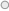Wordsearch
User Rating:/ 0
PoorBest

V  Y  S  D  C  V  B  K  T  H  G  B  W  X  T  D  L

B  T  O  R  V  H  G  N  G  N  F  W  R  H  N  L  Q

B  Z  N  K  X  G  I  K  T  R  X  G  W  T  Z  Q  W

O  D  G  M  J  A  M  Y  C  T  I  N  N  E  Z  E  H

H  S  S  G  V  K  G  X  M  O  G  I  H  P  A  A  K

F  T  F  Z  Q  X  T  L  A  T  R  N  S  Y  J  Q  J

J  Z  R  C  I  K  S  G  Z  Z  E  E  R  G  L  M  U

P  Q  O  O  A  Y  F  G  H  R  G  T  X  A  R  V  D

P  Z  M  U  J  G  U  W  X  Q  D  S  Q  P  D  U  L

V  P  T  N  E  J  X  U  U  U  C  I  T  R  C  I  R

Z  T  H  T  Z  I  V  V  V  H  Z  L  P  R  V  B  O

C  P  E  R  Z  O  O  Q  R  G  S  Y  Q  L  K  U  P

Y  H  S  Y  F  M  S  O  N  Z  J  S  F  Y  M  C  N

U  B  H  K  O  S  R  E  H  S  U  A  A  U  Z  S  E

S  M  O  I  U  S  K  I  U  A  H  E  S  X  N  L  V

N  I  W  D  U  J  B  S  O  M  F  I  Z  O  D  T  D

F  D  S  I  V  S  Z  L  U  L  C  W  N  U  Q  F  K

 country easylistening jazz music radio rock songsfromtheshows

### On Air Now ...

Steve JowleSteve plays your requests and dedications.